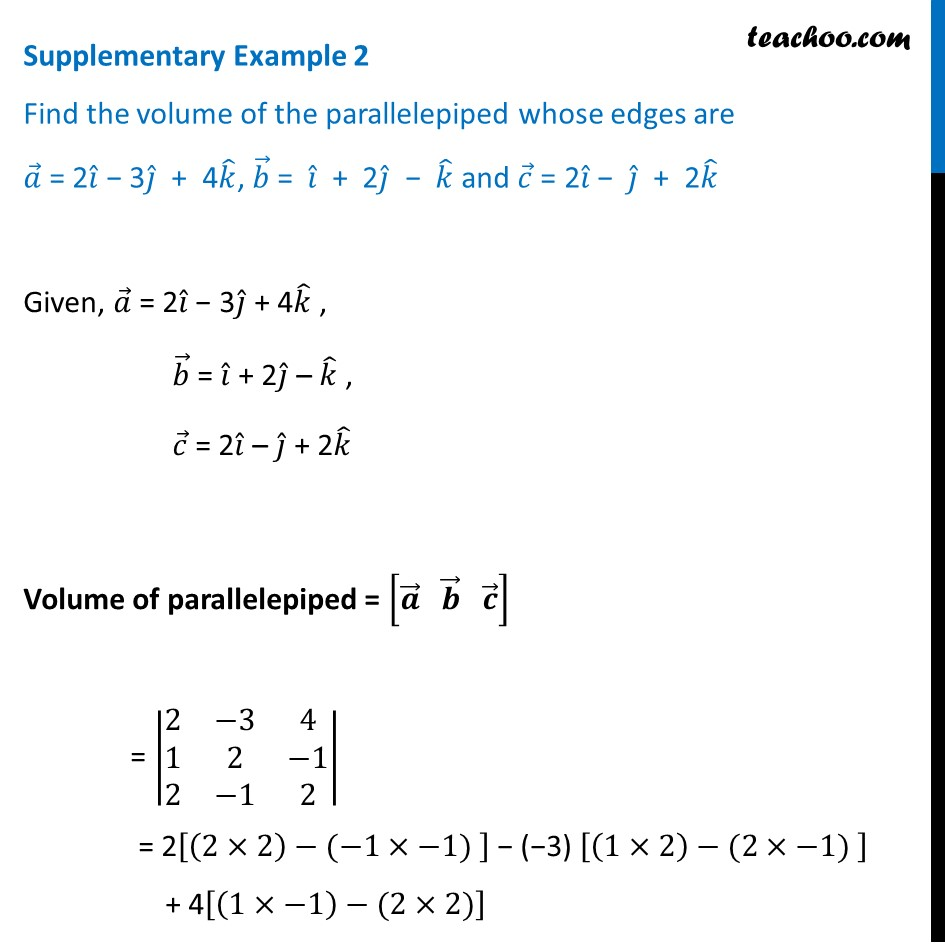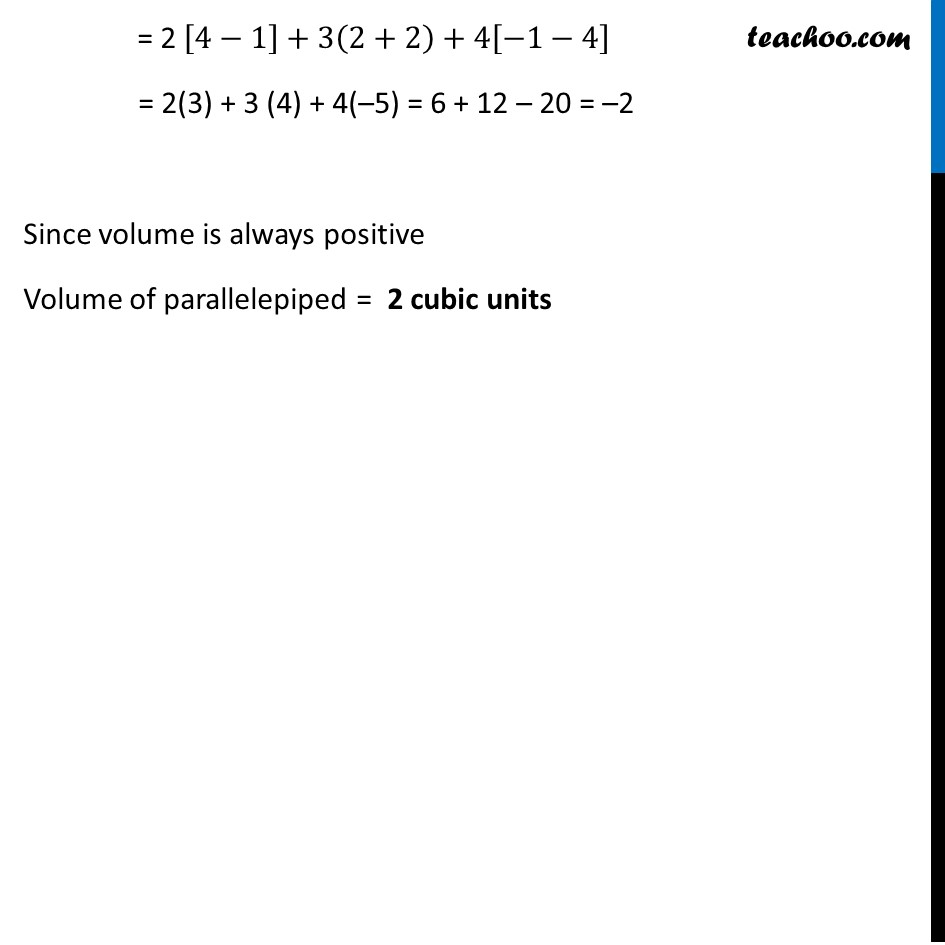Supplementary examples and questions from CBSE

Chapter 10 Class 12 Vector Algebra
Serial order wiseLearn in your speed, with individual attention - Teachoo Maths 1-on-1 Class

### Transcript

Supplementary Example 2 Find the volume of the parallelepiped whose edges are 𝑎 ⃗ = 2𝑖 ̂ − 3𝑗 ̂ + 4𝑘 ̂, 𝑏 ⃗ = 𝑖 ̂ + 2𝑗 ̂ − 𝑘 ̂ and 𝑐 ⃗ = 2𝑖 ̂ − 𝑗 ̂ + 2𝑘 ̂ Given, 𝑎 ⃗ = 2𝑖 ̂ − 3𝑗 ̂ + 4𝑘 ̂ , 𝑏 ⃗ = 𝑖 ̂ + 2𝑗 ̂ – 𝑘 ̂ , 𝑐 ⃗ = 2𝑖 ̂ – 𝑗 ̂ + 2𝑘 ̂ Volume of parallelepiped = [𝒂 ⃗" " 𝒃 ⃗" " 𝒄 ⃗ ] = |■8(2&−3&4@1&2&−1@2&−1&2)| = 2[(2×2)−(−1×−1) ] − (−3) [(1×2)−(2×−1) ] + 4[(1×−1)−(2×2)] = 2 [4−1]+3(2+2)+4[−1−4] = 2(3) + 3 (4) + 4(–5) = 6 + 12 – 20 = –2 Since volume is always positive Volume of parallelepiped = 2 cubic units